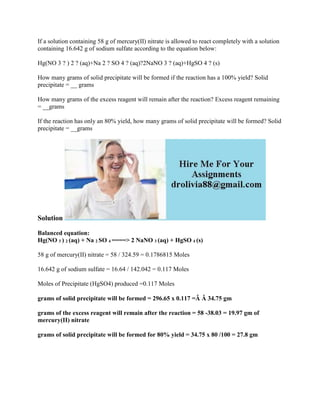Seu SlideShare está sendo baixado. ×

# If a solution containing 58 g of mercury(II) nitrate is allowed to rea.docx

Anúncio
Anúncio
Anúncio
Anúncio
Anúncio
Anúncio
Anúncio
Anúncio
Anúncio
Anúncio
AnúncioCarregando em…3
×

1 de 2 Anúncio

# If a solution containing 58 g of mercury(II) nitrate is allowed to rea.docx

If a solution containing 58 g of mercury(II) nitrate is allowed to react completely with a solution containing 16.642 g of sodium sulfate according to the equation below:
Hg(NO 3 ? ) 2 ? (aq)+Na 2 ? SO 4 ? (aq)?2NaNO 3 ? (aq)+HgSO 4 ? (s)
How many grams of solid precipitate will be formed if the reaction has a 100% yield? Solid precipitate = __ grams
How many grams of the excess reagent will remain after the reaction? Excess reagent remaining = __grams
If the reaction has only an 80% yield, how many grams of solid precipitate will be formed? Solid precipitate = __grams
Solution
Balanced equation:
Hg(NO 3 ) 2 (aq) + Na 2 SO 4 ====> 2 NaNO 3 (aq) + HgSO 4 (s)
58 g of mercury(II) nitrate = 58 / 324.59 = 0.1786815 Moles
16.642 g of sodium sulfate = 16.64 / 142.042 = 0.117 Moles
Moles of Precipitate (HgSO4) produced =0.117 Moles
grams of solid precipitate will be formed = 296.65 x 0.117 =Â Â 34.75 gm
grams of the excess reagent will remain after the reaction = 58 -38.03 = 19.97 gm of mercury(II) nitrate
grams of solid precipitate will be formed for 80% yield = 34.75 x 80 /100 = 27.8 gm
.

If a solution containing 58 g of mercury(II) nitrate is allowed to react completely with a solution containing 16.642 g of sodium sulfate according to the equation below:
Hg(NO 3 ? ) 2 ? (aq)+Na 2 ? SO 4 ? (aq)?2NaNO 3 ? (aq)+HgSO 4 ? (s)
How many grams of solid precipitate will be formed if the reaction has a 100% yield? Solid precipitate = __ grams
How many grams of the excess reagent will remain after the reaction? Excess reagent remaining = __grams
If the reaction has only an 80% yield, how many grams of solid precipitate will be formed? Solid precipitate = __grams
Solution
Balanced equation:
Hg(NO 3 ) 2 (aq) + Na 2 SO 4 ====> 2 NaNO 3 (aq) + HgSO 4 (s)
58 g of mercury(II) nitrate = 58 / 324.59 = 0.1786815 Moles
16.642 g of sodium sulfate = 16.64 / 142.042 = 0.117 Moles
Moles of Precipitate (HgSO4) produced =0.117 Moles
grams of solid precipitate will be formed = 296.65 x 0.117 =Â Â 34.75 gm
grams of the excess reagent will remain after the reaction = 58 -38.03 = 19.97 gm of mercury(II) nitrate
grams of solid precipitate will be formed for 80% yield = 34.75 x 80 /100 = 27.8 gm
.

Anúncio
Anúncio

### If a solution containing 58 g of mercury(II) nitrate is allowed to rea.docx

1. 1. If a solution containing 58 g of mercury(II) nitrate is allowed to react completely with a solution containing 16.642 g of sodium sulfate according to the equation below: Hg(NO 3 ? ) 2 ? (aq)+Na 2 ? SO 4 ? (aq)?2NaNO 3 ? (aq)+HgSO 4 ? (s) How many grams of solid precipitate will be formed if the reaction has a 100% yield? Solid precipitate = __ grams How many grams of the excess reagent will remain after the reaction? Excess reagent remaining = __grams If the reaction has only an 80% yield, how many grams of solid precipitate will be formed? Solid precipitate = __grams Solution Balanced equation: Hg(NO 3 ) 2 (aq) + Na 2 SO 4 ====> 2 NaNO 3 (aq) + HgSO 4 (s) 58 g of mercury(II) nitrate = 58 / 324.59 = 0.1786815 Moles 16.642 g of sodium sulfate = 16.64 / 142.042 = 0.117 Moles Moles of Precipitate (HgSO4) produced =0.117 Moles grams of solid precipitate will be formed = 296.65 x 0.117 =Â Â 34.75 gm grams of the excess reagent will remain after the reaction = 58 -38.03 = 19.97 gm of mercury(II) nitrate grams of solid precipitate will be formed for 80% yield = 34.75 x 80 /100 = 27.8 gm